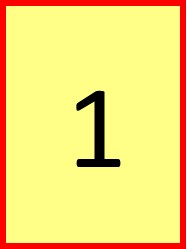07899 756585

# House of Maths School Workshops Primary & Secondary in Dorset & South - WHAT’S SPECIAL ABOUT THE NUMBER ONE?### WHAT’S INTERESTING ABOUT THE NUMBER ONE?

ONE is the only number that’s the same in binary, base 10 and Roman Numerals.

It’s the wheels on a unicycle, the rails on a monorail and the players when you go solo.

One is the first odd number, the first triangular, square, pentagonal and hexagonal number, and the first tetrahedralcube and Fibonacci number.

It’s the only whole number whose letters O-N-E are in reverse alphabetical order (but which is the only whole number whose letters are in forward alphabetical order?).

One is the only positive integer (whole number) which is neither prime (exactly two factors: one and itself) nor composite (more than two factors).

NORMAL ARITHMETIC:
The number one is used to make up all the other integers:
1=1
2=1+1
3=1+1+1 etc

AXIOMATIC SET THEORY (von Neumann ordinals) :
The number zero is used to make up all the other integers:
0=∅={} (the empty set)
1= {Ø}={0}
2={Ø, {Ø}}={0,{0}}
3={Ø,{Ø},{Ø, {Ø}}}={0,{0},{0,{0}}} etc

Axiomatic set theory started in the early 1900’s as an attempt to place mathematics on a firm logical footing free of paradoxes. For further reading, search Wikipedia for Russell’s Paradox, Cantor’s theorem, cardinal numbers, ordinal numbers, put aside about a month of reading time and get ready for an incredible ride!
Which definition of the integers do you prefer: using ones or zeroes?

One is gender-neutral: one is so pleased you are reading one’s post!

So. number one, we salute you: you are singularly unique and one of a kind. A big hand for NUMBER ONE!